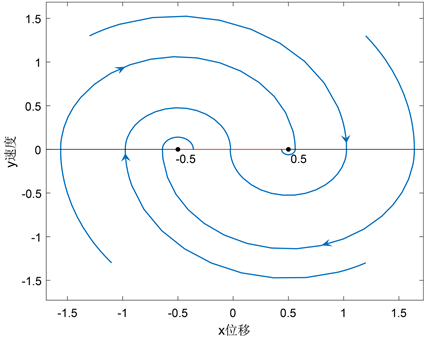# 平面库伦摩擦系统的动力学分析Dynamic Analysis of Planar Coulomb Friction System

DOI: 10.12677/AAM.2021.104143, PDF, HTML, XML, 下载: 8  浏览: 23

Abstract: The existence of Coulomb friction in mechanical system leads to the existence of discontinuity. In this paper, the global dynamic behavior of an object moving on a horizontal plane under Coulomb friction is studied. In this case, the points on the sliding mode domain of the discontinuous system are all pseudo-equilibrium points of the system. On this basis, by constructing the Poincaré mapping, the conclusion that the set of points in the sliding mode domain is the global attractor of the system is given. Finally, the conclusion that the attractor is convergent in finite time is proved by numerical simulation.

1. 引言

2. 系统数学模型的建立Figure 1. Coulomb friction system

$m\stackrel{¨}{x}+p\left(\stackrel{˙}{x}\right)+kx=0$ (1.1)

$p\left(\stackrel{˙}{x}\right)=\left\{\begin{array}{l}{p}_{0},\text{}\stackrel{˙}{x}>0\hfill \\ -{p}_{0},\text{}\stackrel{˙}{x}<0\hfill \end{array}$ (1.2)

${p}_{0}\ne 0$ 时，由于系统(1.1)~(1.2)是一个右端不连续的微分系统，当考虑到系统(1.1)在Filippov意义下的解，不难知道系统(1.1)的解是存在的，且满足初值条件 $x\left(0\right)={x}_{0},y\left(0\right)={y}_{0},$ 存在区间为 $\left[0,+\infty \right)$，并且系统的解是右唯一的。为了后续的方便讨论，将系统(1.1)~(1.2)化为等价的平面微分系统，令 $\stackrel{˙}{x}=y$

$\left\{\begin{array}{l}\stackrel{˙}{x}=y\hfill \\ \stackrel{˙}{y}=-\frac{kx}{m}-\frac{p\left(y\right)}{m}\hfill \end{array}$ (1.3)

$p\left(y\right)=\left\{\begin{array}{l}{p}_{0},\text{}y>0\hfill \\ -{p}_{0},\text{}y<0\hfill \end{array}$ (1.4)

$\left\{\begin{array}{l}\stackrel{˙}{x}=y\hfill \\ \stackrel{˙}{y}=-\frac{kx}{m}-\frac{{p}_{0}}{m},\text{}y>0\hfill \end{array}$ (1.5)

$\left\{\begin{array}{l}\stackrel{˙}{x}=y\hfill \\ \stackrel{˙}{y}=-\frac{kx}{m}+\frac{{p}_{0}}{m},\text{}y<0\hfill \end{array}$ (1.6)

$m{y}^{2}+k{\left(x+\frac{{p}_{0}}{k}\right)}^{2}={c}_{1}$ (1.7)

$m{y}^{2}+k{\left(x-\frac{{p}_{0}}{k}\right)}^{2}={c}_{2}$ (1.8)

3. 切换线上滑模动力学行为Figure 2. Vector field distribution

${\Sigma }_{{C}_{1}}=\left\{\left(x,y\right)|-{A}_{1}

${\Sigma }_{s}=\left\{\left(x,y\right)|-\frac{{p}_{0}}{k}

${\Sigma }_{{C}_{2}}=\left\{\left(x,y\right)|\frac{{p}_{0}}{k}

$\left\{\begin{array}{c}\stackrel{˙}{x}=y,\\ \stackrel{˙}{y}=0,\end{array}$ (2.1)

4. 系统的全局动力学行为

${P}_{L}\left({x}_{0}\right)=\left\{\begin{array}{l}{x}_{1}^{L},{x}_{0}\in \left(\frac{{p}_{0}}{k},{A}_{2}\right]\hfill \\ {x}_{0},{x}_{0}=\left[-{A}_{1},\frac{{p}_{0}}{k}\right]\hfill \end{array}$

${P}_{U}\left({x}_{0}\right)=\left\{\begin{array}{l}{x}_{1}^{U},{x}_{0}\in \left[-{A}_{1},-\frac{{p}_{0}}{k}\right)\hfill \\ {x}_{0},{x}_{0}=\left[\frac{{p}_{0}}{k},-{A}_{2}\right]\hfill \end{array}$

${x}_{1}^{U}={P}_{U}\left({x}_{0}\right)=-{x}_{0}-\frac{2{p}_{0}}{k},\text{}{x}_{0}\in \left[-{A}_{1},-\frac{{p}_{0}}{k}\right)$ (3.1)

${x}_{1}^{L}={P}_{L}\left({x}_{0}\right)=-{x}_{0}+\frac{2{p}_{0}}{k},\text{}{x}_{0}\in \left(\frac{{p}_{0}}{k},{A}_{2}\right]$ (3.2)

$P\left({x}_{0}\right)={P}_{U}\left({P}_{L}\left({x}_{0}\right)\right)={x}_{0}-\frac{4{p}_{0}}{k},\text{}{x}_{0}\in \left[\frac{3{p}_{0}}{k},{A}_{2}\right]$ (3.3)

$P\left({x}_{0}\right)\in {\Sigma }_{S},\text{}这里{P}^{N}=\underset{N}{\underset{︸}{P\circ P\circ \cdots \circ P}}$

5. 数值仿真及应用分析

$k=1,\text{}m=1,\text{}{p}_{0}=\frac{1}{2}$ 时，系统的滑模域区间为 $\left[-0.5,0.5\right]$，从图3中可以看出，在有限时间内从任何点出发的轨线，最后都收敛到 $\left[-0.5,0.5\right]$ 区间的滑模域上。Figure 3. Trajectory phase diagram of plane Coulomb friction system

6. 结论

  Urbakh, M., Klafter, J., Gourdon, D., et al. (2004) The Nonlinear Nature of Friction. Nature, 430, 525. https://doi.org/10.1038/nature02750  王松年. 摩擦学原理及应用[M]. 北京: 中国铁道出版社, 1990.  Berger, E. (2002) Friction Modeling for Dynamic System Simulation. Applied Mechanics Reviews, 55, 25-32. https://doi.org/10.1115/1.1501080  Bacciotti, A. and Ceragioli. F. (1999) Stability and Stabilization of Discontinuous Systems and Nonsmooth Lyapunov Functions. ESAIM Control Optimisation and Calculus of Variations, 4, 361-376. https://doi.org/10.1051/cocv:1999113  Leine, R.I. and Campen, D. (2006) Bifurcation Phenomena in Non-Smooth Dynamical Systems. European Journal of Mechanics—A/Solids, 25, 595-616. https://doi.org/10.1016/j.euromechsol.2006.04.004  Alvarez, J., Orlov, I. and Acho, L. (2000) An Invariance Principle for Discontinuous Dynamic Systems with Application to a Coulomb Friction Oscillator. Journal of Dynamic Systems Measurement and Control, 122, 317-322. https://doi.org/10.1115/1.1317229  黄立宏, 郭振远, 王佳伏. 右端不连续微分方程理论与应用[M]. 北京: 科学出版社, 2011.  丁千, 翟红梅. 机械系统摩擦动力学研究进展[J]. 力学进展, 2013, 43(1): 112-131.